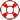Standard:

Description:

Standard:

Description:

Standard:

Description:

Standard:

# Math.4.MD.7 or 4.MD.C.7

Description:

## Recognize angle measure as additive. When an angle is decomposed into non-overlapping parts, the angle measure of the whole is the sum of the angle measures of the parts. Solve addition and subtraction problems to find unknown angles on a diagram in real world and mathematical problems, e.g., by using an equation with a symbol for the unknown angle measure.4th Grade Math - Angles Lesson